# Resources tagged with: Multiplication & division

Filter by: Content type:
Age range:
Challenge level:

### There are 153 results

Broad Topics > Calculations and Numerical Methods > Multiplication & division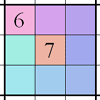### Integrated Product Sudoku

##### Age 11 to 16Challenge Level

This Sudoku puzzle can be solved with the help of small clue-numbers on the border lines between pairs of neighbouring squares of the grid.### The Remainders Game

##### Age 7 to 14Challenge Level

Play this game and see if you can figure out the computer's chosen number.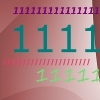### Ones Only

##### Age 11 to 14Challenge Level

Find the smallest whole number which, when mutiplied by 7, gives a product consisting entirely of ones.### One O Five

##### Age 11 to 14Challenge Level

You can work out the number someone else is thinking of as follows. Ask a friend to think of any natural number less than 100. Then ask them to tell you the remainders when this number is divided by. . . .### What Two ...?

##### Age 7 to 11 ShortChallenge Level

56 406 is the product of two consecutive numbers. What are these two numbers?### Tom's Number

##### Age 7 to 11Challenge Level

Work out Tom's number from the answers he gives his friend. He will only answer 'yes' or 'no'.### Product Sudoku

##### Age 11 to 16Challenge Level

The clues for this Sudoku are the product of the numbers in adjacent squares.### Multiples Sudoku

##### Age 11 to 14Challenge Level

Each clue in this Sudoku is the product of the two numbers in adjacent cells.### A First Product Sudoku

##### Age 7 to 14Challenge Level

Given the products of adjacent cells, can you complete this Sudoku?### More Magic Potting Sheds

##### Age 11 to 14Challenge Level

The number of plants in Mr McGregor's magic potting shed increases overnight. He'd like to put the same number of plants in each of his gardens, planting one garden each day. How can he do it?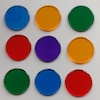### Dicey Array

##### Age 5 to 11Challenge Level

Watch the video of this game being played. Can you work out the rules? Which dice totals are good to get, and why?### Times Right

##### Age 11 to 16Challenge Level

Using the digits 1, 2, 3, 4, 5, 6, 7 and 8, mulitply a two two digit numbers are multiplied to give a four digit number, so that the expression is correct. How many different solutions can you find?### What Is Ziffle?

##### Age 7 to 11Challenge Level

Can you work out what a ziffle is on the planet Zargon?### Surprising Split

##### Age 7 to 11Challenge Level

Does this 'trick' for calculating multiples of 11 always work? Why or why not?### Powerful Factorial

##### Age 11 to 14Challenge Level

6! = 6 x 5 x 4 x 3 x 2 x 1. The highest power of 2 that divides exactly into 6! is 4 since (6!) / (2^4 ) = 45. What is the highest power of two that divides exactly into 100!?### Eminit

##### Age 11 to 14Challenge Level

The number 8888...88M9999...99 is divisible by 7 and it starts with the digit 8 repeated 50 times and ends with the digit 9 repeated 50 times. What is the value of the digit M?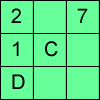### Alphabetti Sudoku

##### Age 11 to 16Challenge Level

This Sudoku requires you to do some working backwards before working forwards.### Factoring Factorials

##### Age 11 to 14Challenge Level

Find the highest power of 11 that will divide into 1000! exactly.### Multiply the Addition Square

##### Age 11 to 14Challenge Level

If you take a three by three square on a 1-10 addition square and multiply the diagonally opposite numbers together, what is the difference between these products. Why?### Code Breaker

##### Age 7 to 11Challenge Level

This problem is based on a code using two different prime numbers less than 10. You'll need to multiply them together and shift the alphabet forwards by the result. Can you decipher the code?### Why 8?

##### Age 11 to 14Challenge Level

Choose any four consecutive even numbers. Multiply the two middle numbers together. Multiply the first and last numbers. Now subtract your second answer from the first. Try it with your own. . . .### Missing Multipliers

##### Age 7 to 14Challenge Level

What is the smallest number of answers you need to reveal in order to work out the missing headers?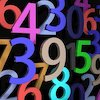### Countdown

##### Age 7 to 14Challenge Level

Here is a chance to play a version of the classic Countdown Game.### Funny Factorisation

##### Age 11 to 16Challenge Level

Using the digits 1 to 9, the number 4396 can be written as the product of two numbers. Can you find the factors?### It Figures

##### Age 7 to 11Challenge Level

Suppose we allow ourselves to use three numbers less than 10 and multiply them together. How many different products can you find? How do you know you've got them all?### Magic Potting Sheds

##### Age 11 to 14Challenge Level

Mr McGregor has a magic potting shed. Overnight, the number of plants in it doubles. He'd like to put the same number of plants in each of three gardens, planting one garden each day. Can he do it?### Thirty Six Exactly

##### Age 11 to 14Challenge Level

The number 12 = 2^2 × 3 has 6 factors. What is the smallest natural number with exactly 36 factors?### Oh! Hidden Inside?

##### Age 11 to 14Challenge Level

Find the number which has 8 divisors, such that the product of the divisors is 331776.### Repeaters

##### Age 11 to 14Challenge Level

Choose any 3 digits and make a 6 digit number by repeating the 3 digits in the same order (e.g. 594594). Explain why whatever digits you choose the number will always be divisible by 7, 11 and 13.### The Genie in the Jar

##### Age 11 to 14Challenge Level

This jar used to hold perfumed oil. It contained enough oil to fill granid silver bottles. Each bottle held enough to fill ozvik golden goblets and each goblet held enough to fill vaswik crystal. . . .### Divide it Out

##### Age 7 to 11Challenge Level

What is the lowest number which always leaves a remainder of 1 when divided by each of the numbers from 2 to 10?### Remainders

##### Age 7 to 14Challenge Level

I'm thinking of a number. My number is both a multiple of 5 and a multiple of 6. What could my number be?### Countdown Fractions

##### Age 11 to 16Challenge Level

Here is a chance to play a fractions version of the classic Countdown Game.### Multiplication Series: Illustrating Number Properties with Arrays

##### Age 5 to 11

This article for teachers describes how modelling number properties involving multiplication using an array of objects not only allows children to represent their thinking with concrete materials,. . . .### Ducking and Dividing

##### Age 7 to 11Challenge Level

Your vessel, the Starship Diophantus, has become damaged in deep space. Can you use your knowledge of times tables and some lightning reflexes to survive?### Gabriel's Problem

##### Age 11 to 14Challenge Level

Gabriel multiplied together some numbers and then erased them. Can you figure out where each number was?### Largest Product

##### Age 11 to 14Challenge Level

Which set of numbers that add to 10 have the largest product?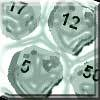### Asteroid Blast

##### Age 7 to 11Challenge Level

A game for 2 people. Use your skills of addition, subtraction, multiplication and division to blast the asteroids.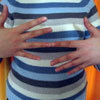### What Is the Question?

##### Age 11 to 16Challenge Level

These pictures and answers leave the viewer with the problem "What is the Question". Can you give the question and how the answer follows?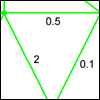### Route Product

##### Age 7 to 11Challenge Level

Find the product of the numbers on the routes from A to B. Which route has the smallest product? Which the largest?### A-magical Number Maze

##### Age 7 to 11Challenge Level

This magic square has operations written in it, to make it into a maze. Start wherever you like, go through every cell and go out a total of 15!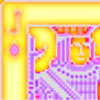### Wild Jack

##### Age 7 to 11Challenge Level

A game for 2 or more players with a pack of cards. Practise your skills of addition, subtraction, multiplication and division to hit the target score.### Zargon Glasses

##### Age 7 to 11Challenge Level

Zumf makes spectacles for the residents of the planet Zargon, who have either 3 eyes or 4 eyes. How many lenses will Zumf need to make all the different orders for 9 families?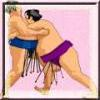### Sumo

##### Age 7 to 11Challenge Level

A number game requiring a strategy.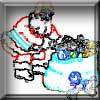### Clever Santa

##### Age 7 to 11Challenge Level

All the girls would like a puzzle each for Christmas and all the boys would like a book each. Solve the riddle to find out how many puzzles and books Santa left.### Being Resilient - Primary Number

##### Age 5 to 11Challenge Level

Number problems at primary level that may require resilience.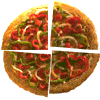### Fair Feast

##### Age 5 to 11Challenge Level

Here is a picnic that Petros and Michael are going to share equally. Can you tell us what each of them will have?### Rabbits in the Pen

##### Age 7 to 11Challenge Level

Using the statements, can you work out how many of each type of rabbit there are in these pens?### Can You Make 100?

##### Age 11 to 14Challenge Level

How many ways can you find to put in operation signs (+ - x Ã·) to make 100?### X Is 5 Squares

##### Age 7 to 11Challenge Level

Can you arrange 5 different digits (from 0 - 9) in the cross in the way described?